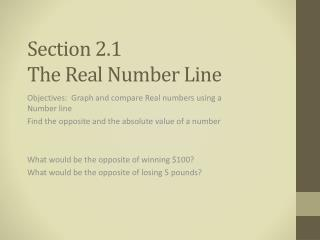DownloadDownload PresentationSection 2.1 The Real Number Line

Section 2.1 The Real Number Line

Télécharger la présentationSection 2.1 The Real Number Line

- - - - - - - - - - - - - - - - - - - - - - - - - - - E N D - - - - - - - - - - - - - - - - - - - - - - - - - - -
Presentation Transcript

1. Section 2.1The Real Number Line Objectives: Graph and compare Real numbers using a Number line Find the opposite and the absolute value of a number What would be the opposite of winning \$100? What would be the opposite of losing 5 pounds?

2. Definitions • Real Numbers: the set of numbers consisting of positive numbers, the negative numbers, and zero • Real Number Line: a line that pictures real numbers as points • Origin of a Number Line: the point labeled zero on a number line • Positive numbers: any of the numbers greater than zero • Negative numbers: any of the numbers less than zero • Integers: any of the numbers either negative, zero, or positive without decimals or fractions • Opposites: two points on a number line that are the same distance from the origin but are on opposite sides of it • Absolute value: the distance between the origin and the point representing the real number • Counterexample: an example used to show that a given statement is false

3. EX 1: Graph the numbers on a number line. Then write two inequalities that compare the two numbers • a. • b. EX 2: Write the following numbers in increasing order:

4. EX 3: Find the opposite of the number a. -3 b. 0 c. 5/6 d. -1.2 EX 4: Evaluate the expression a. b. c.

5. EX 5: Use the table which lists several players and their final scores at a 1998 Ladies Professional Golf tournament.In golf the total score is given as the number of strokes above or below par, the expected score. If you are at “even par”, your score is zero. The player with the lowest score wins. • Which player scored closest to par? • Which player scored farther from par? • Which players scored above par? • Which player scored below par? • Which player won the tournament?

6. EX 6: Decide whether the statement is true or false. If it is false, give a counterexample • A) The expression is always positive. • B) The symbol is sometimes equal to • C) The absolute value of a number is always greater than the number.

7. Assignment:p. 67 18 – 45x3, 44; 48 – 56 evens, 62 – 70 evens, 90 – 102x3